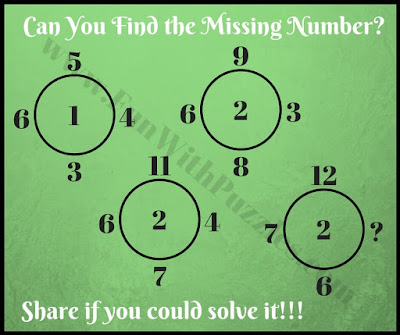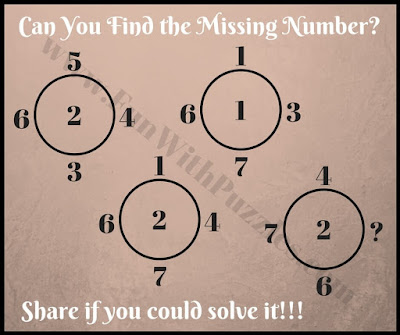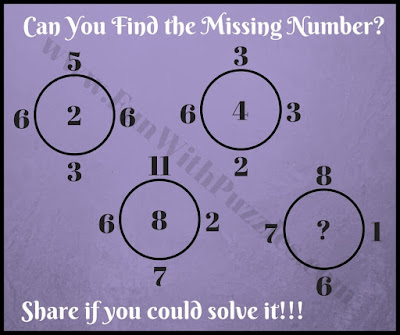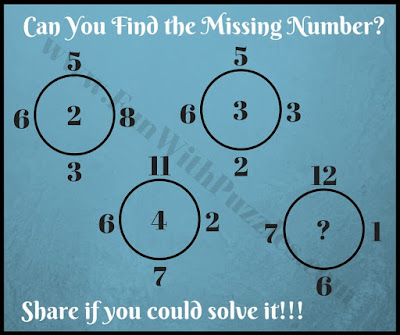There are many Maths Brain Teasers on this website. These Maths Brain Teasers ranges from the types of Series Math Puzzles in which one has to find the next number in the series to Maths Equations Puzzles in which one has to find calculate the value of a particular shape or symbol while solving maths equations. Also, there many Maths Brain Teasers which contains numbers around a shape and one has to find the missing number. Today's Math Brainteasers are based upon Circle shape. These puzzles are an extension of earlier published similar puzzles titled "Tricky Maths Brain Challenges with answers".
In each of these Maths Brain Teasers, five numbers are given in and around the circle. These numbers share some relationship with each other. One has to find this relationship and then solve it for the missing number which will replace the question mark.
The answer to these Maths Brain Teasers is given at the end. Do post your answers along with an explanation in the comments section.1. Clever thinking maths question2. Maths picture puzzle3. Mind breaking math circle puzzle brain teaser4. Simple math circle puzzle brain teaser5. Tricky maths picture puzzle

The answer to these Maths Brain Teasers are as below

A1. 5

A2. 5

A3. 8

A4. 6

A5. 9